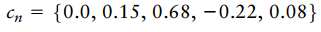Create an Account

Home / Questions / The sampled impulse response of a data transmission system encompassing the transmit filte...

The sampled impulse response of a data transmission system encompassing the transmit filter and channel is defined by For zero forcing equalization of the system it is proposed to use a three

The sampled impulse response of a data-transmission system (encompassing the transmit filter and channel) is defined byFor zero-forcing equalization of the system, it is proposed to use a three-tap transversal filter.

(a) Calculate the adjustable weights of the equalizer.

(b) Using the equalizer determined in part (a), calculate the residual intersymbol interference at the equalizer output.

(c) Identify the magnitude of the sample making the largest contribution to the residual intersymbol interference.

Jul 30 2020 View more View LessSubscribe To Get Solution